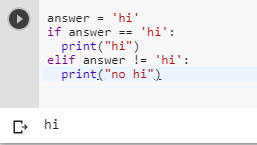2 views
in Python

How would you say does not equal?

Like

if hi == hi:

print "hi"

elif hi (does not equal) bye:

print "no hi"

Is there something equivalent to == that means "not equal"?

closed

by (106k points)
edited by

Yes, there a “not equal” operator in Python which is denoted by !=, if you want to compare object identity, you can use the keyword is and its negation is not.

Below is your code with the != operator:-

if answer == 'hi':

print("hi")

elif answer != 'hi':

print("no hi")To know more about this you can have a look at the following video tutorial:-

+1 vote